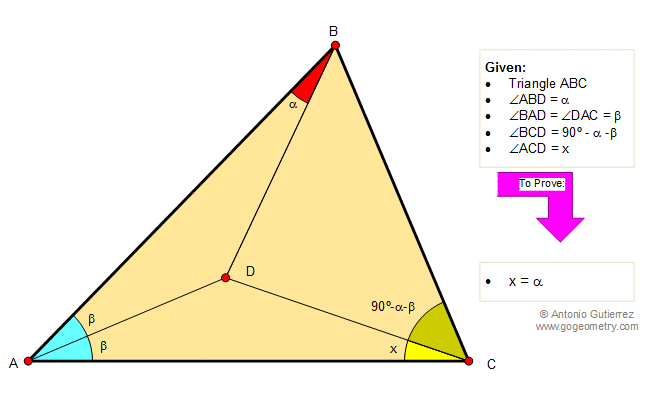# Geometry Problem 105. Angles, Triangle, Interior Point. Level: High School, Honors Geometry, College, Mathematics Education

 In the figure below, given a triangle ABC, angle ABD = a , angle BAD = angle DAC = b , angle BCD = 90 - a - b , and angle ACD = x. Prove that x = a. View or post a solution..
 Home | Search | Problems | 101-110 | Email | View or post a solution | by Antonio Gutierrez